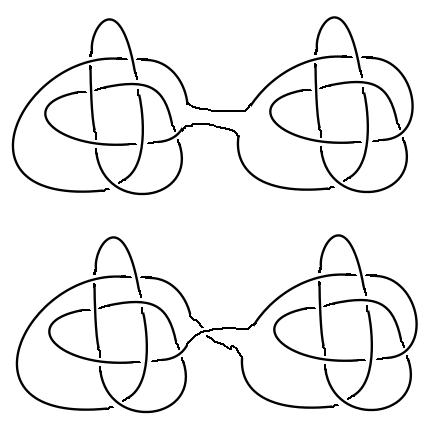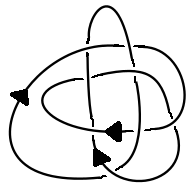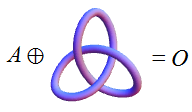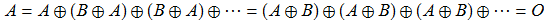# Eilenberg-Mazur swindle

The connect sum of two knots is obtained by joining them together in the obvious way. There is, however, one small detail. If you define this naïvely, then you won’t necessarily obtain an unambiguous connect sum. For instance, consider the following:It’s difficult to show that the two above knots are non-equivalent (as usual), but it is indeed the case. The best way to avoid this problem is to consider directed knots instead of undirected knots. The knot shown below is one of the simplest ‘non-invertible knot’, i.e. one which cannot be deformed into itself such that the directions of the arrows change:The first example can be viewed as the sum of two equally directed knots, whereas the second example is the sum of two oppositely directed knots. Now that we’ve imposed direction, the connect sum of knots is well-defined; I’ll leave proving it as a straightforward exercise for the reader.

Note that connect sum is both commutative and associative, with the unknot O (simple loop) forming an identity. The set of knots under the operation of connect sum is thus an Abelian monoid.For this to be an Abelian group, we would need inverses. Unfortunately, this is not the case; for instance, there is no knot A that behaves as an ‘anti-trefoil’:A simple proof of this is to note that the trefoil knot is tricolourable, whereas the unknot is not, and that the connect sum of a tricolourable knot and an arbitrary knot gives another tricolourable knot. This contradicts the above equation, so there is no anti-trefoil. Indeed, more generally, there are no two non-trivial knots A and B such that$A \oplus B = O$. The standard proof is the Mazur swindle:This appears to be massively cheating. For instance, the argument could be applied to 1 and −1 to deduce that 1 = 0, which is clearly erroneous. However, it is intuitive that this reasoning works in the context of knots, since it can be realised geometrically.

Interestingly, the Abelian monoid of knots exhibits unique factorisation (Schubert’s theorem), so it suffices to categorise the ‘prime’ knots that cannot be expressed as connect sums. The knots tabulated by Rolfsen were the prime knots, the first few of which can be viewed here.

### Other applications

In addition to knot theory, the principle of the Mazur swindle can be applied to other objects. For instance, we can express the free module$\mathbb{Z}_6^{\infty}$ as the direct sum$\mathbb{Z}_2 \oplus \mathbb{Z}_6^{\infty}$ of itself and something which isn’t a free module over$\mathbb{Z}_6$. Without this idea, it’s pretty difficult to find modules satisfying these properties.

This entry was posted in Uncategorized. Bookmark the permalink.

### 0 Responses to Eilenberg-Mazur swindle

1.Alexei Averchenko says:

So what is it, then, if not cheating? It seems that we need a topology on the monoid in order to make this precise. Also, does this monoid have cancellation property?

•apgoucher says:

A knot is formally defined as a homeomorphism between the circle S_1 and a subset of R^3. It’s possible to define the connect sum of two knots without even viewing the set of distinct knots as a monoid. The geometric definition extends to a connect sum of countably many knots.

I’m not sure how you would define a topology on the monoid of knots. Which sets of knots would you consider to be open sets?